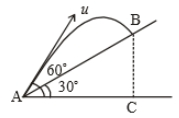A ball rolls off top of a staircase with a horizontal velocity u . If the steps are h metre high and b mere wide, the ball will just hit the edge of nth step if n equals to

1. $\frac{h{u}^{2}}{g{b}^{2}}$

2. $\frac{{u}^{2}8}{g{b}^{2}}$

3. $\frac{2h{u}^{2}}{g{b}^{2}}$

4. $\frac{2{u}^{2}g}{h{b}^{2}}$

Subtopic:  Uniformly Accelerated Motion |
Please attempt this question first.

Difficulty Level:

A man standing on the roof of a house of height h throws one particle vertically downwards and another particle horizontally with the same velocity u. The ratio of their velocities when they reach the earth’s surface will be

1.

2. 1:2

3. 1:1

4.

Subtopic:  Uniformly Accelerated Motion |
Please attempt this question first.

Difficulty Level:

A particle is projected at an angle $\theta$ with horizontal with an initital speed u. When it makes an angle $\alpha$ with horizontal, its speed v is-

1. $u\mathrm{cos}\theta$

2.

3. $\frac{u\mathrm{sin}\theta }{\mathrm{sin}\alpha }$

4. $\frac{u\mathrm{cos}\theta }{\mathrm{cos}\alpha }$

Subtopic:  Projectile Motion |
To view explanation, please enrol in the course below.
NEET 2021 - Achiever Batch - Aryan Raj Singh

Difficulty Level:

A body is projected with velocity $20\sqrt{3}$ m/s with an angle of projection 60$°$ with horizontal. Calculate velocity on that point where body makes an angle 30$°$ with the horizontal.

1. 20 m/s

2.

3.

4. 10 m/s

Subtopic:  Projectile Motion |
To view explanation, please enrol in the course below.
NEET 2021 - Achiever Batch - Aryan Raj Singh

Difficulty Level:

A particle is moving with veocity $\stackrel{⇀}{\mathrm{V}}=k\left(y\stackrel{^}{i}+x\stackrel{^}{j}\right)$; where k is constant. The general equation for path is:

1. $\mathrm{y}={\mathrm{x}}^{2}+\mathrm{constant}$

2. ${\mathrm{y}}^{2}={\mathrm{x}}^{2}+\mathrm{constant}$

3. $\mathrm{y}=\mathrm{x}+\mathrm{constant}$

4. xy=constant

Subtopic:  Speed & Velocity |
To view explanation, please enrol in the course below.
NEET 2021 - Achiever Batch - Aryan Raj Singh

Difficulty Level:

A particle is projected with a velocity u making an angle $\mathrm{\theta }$ with the horizontal. At any instant, its velocity v is at right angles to its initial velocity u; then v is:

1.  u cos $\mathrm{\theta }$

2.  u tan $\mathrm{\theta }$

3.  u cot $\mathrm{\theta }$

4.  u sec $\mathrm{\theta }$

Subtopic:  Projectile Motion |
To view explanation, please enrol in the course below.
NEET 2021 - Achiever Batch - Aryan Raj Singh

Difficulty Level:

A projectile is given an initial velocity of $\stackrel{^}{i}+2\stackrel{^}{j}$. The cartesian equation of its path is (g = 10 ${\mathrm{ms}}^{-2}$

1. $\mathrm{y}=2\mathrm{x}-5{\mathrm{x}}^{2}$

2. $\mathrm{y}=\mathrm{x}-5{\mathrm{x}}^{2}$

3. $4\mathrm{y}=2\mathrm{x}-5{\mathrm{x}}^{2}$

4. $\mathrm{y}=2\mathrm{x}-25{\mathrm{x}}^{2}$

Subtopic:  Projectile Motion |
To view explanation, please enrol in the course below.
NEET 2021 - Achiever Batch - Aryan Raj Singh

Difficulty Level:

A ship A is moving westwards with a speed of 10 km ${\mathrm{h}}^{-1}$ and a ship B, 100 km south of A is moving northwards with a speed of 10 km ${\mathrm{h}}^{-1}$. The time after which the distance between them becomes the shortest, is:

1. 5 hr

2. $5\sqrt{2}$ hr

3. $10\sqrt{2}$ hr

4. 0 hr

Subtopic:  Relative Motion |
To view explanation, please enrol in the course below.
NEET 2021 - Achiever Batch - Aryan Raj Singh

Difficulty Level:

Time taken by the projectile to reach from A to B is t. Then the distance AB is equal to :1. $\frac{ut}{\sqrt{3}}$

2. $\frac{\sqrt{3}ut}{2}$

3. $\sqrt{3}ut$

4. 2ut

Subtopic:  Projectile Motion |
To view explanation, please enrol in the course below.
NEET 2021 - Achiever Batch - Aryan Raj Singh

Difficulty Level:

A particle projected with kinetic energy ${\mathrm{k}}_{0}$ with an angle of projection $\mathrm{\theta }$. Then the variation of kinetic K with vertical displacement y is

1.  linear

2.  parabolic

3.  hyperbolic

4.  periodic

Subtopic:  Projectile Motion |
To view explanation, please enrol in the course below.
NEET 2021 - Achiever Batch - Aryan Raj Singh

Difficulty Level: